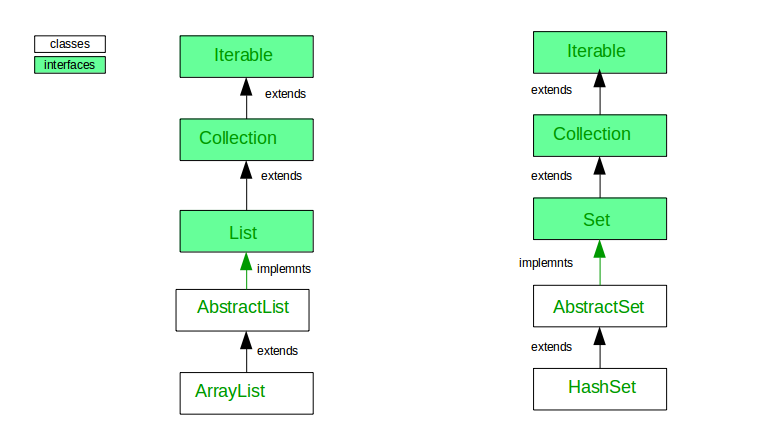# Difference between ArrayList and HashSet in Java

Here are couple of differences between ArrayList and HashSet.

1. Inheritance:2. Implementation:
Implementation : ArrayList implements List interface while HashSet implements Set interface in Java.
3. Internal implementation:
ArrayList is backed by an Array while HashSet is backed by an HashMap.
4. Duplicates :
ArrayList allows duplicate values while HashSet doesn’t allow duplicates values.
5. Constructor :
ArrayList have three constructor which are ArrayList(), ArrayList(int capacity) ArrayList(int Collection c) while HashSet have four constructor which are HashSet(), HashSet(int capacity), HashSet(Collection c) and HashSet(int capacity, float loadFactor)
6. Ordering :
ArrayList maintains the order of the object in which they are inserted while HashSet is an unordered collection and doesn’t maintain any order.
7. Indexing :
ArrayList is index based we can retrieve object by calling get(index) method or remove objects by calling remove(index) method while HashSet is completely object based. HashSet also does not provide get() method.
8. Null Object:
ArrayList not apply any restriction, we can add any number of null value while HashSet allow one null value.
9. Syntax:
ArrayList:-

ArrayList list=new ArrayList();

HashSet:-

HashSet set=new HashSet();

ArrayList example

 `// Java program to demonstrate working of ArrayList in Java ` ` `  `import` `java.io.*; ` `import` `java.util.*; ` ` `  `class` `ArrayListTest { ` ` `  `    ``public` `static` `void` `main(String[] args) ` `        ``throws` `IOException ` `    ``{ ` `        ``// size of ArrayList ` `        ``int` `n = ``5``; ` ` `  `        ``// declaring ArrayList with initial size n ` `        ``List al = ``new` `ArrayList<>(n); ` ` `  `        ``// Appending the new element at the end of the list ` `        ``for` `(``int` `i = ``1``; i <= n; i++) { ` `            ``al.add(i); ` `        ``} ` ` `  `        ``// Printing elements ` `        ``System.out.println(al); ` ` `  `        ``// Remove element at index 3 ` `        ``al.remove(``3``); ` ` `  `        ``// Displaying ArrayList after deletion ` `        ``System.out.println(al); ` ` `  `        ``// Printing elements one by one ` `        ``for` `(``int` `i = ``0``; i < al.size(); i++) { ` `            ``System.out.print(al.get(i) + ``" "``); ` `        ``} ` `    ``} ` `} `

Output:

```[1, 2, 3, 4, 5]
[1, 2, 3, 5]
1 2 3 5
```

HashSet example

 `// Java program to demonstrate working of HashSet ` ` `  `import` `java.util.HashSet; ` `import` `java.util.Set; ` ` `  `class` `HashSetDemo { ` ` `  `    ``public` `static` `void` `main(String[] args) ` `    ``{ ` ` `  `        ``// Create a HashSet ` `        ``Set hs = ``new` `HashSet<>(); ` ` `  `        ``// add elements to HashSet ` `        ``hs.add(``1``); ` `        ``hs.add(``2``); ` `        ``hs.add(``3``); ` `        ``hs.add(``4``); ` ` `  `        ``// Duplicate removed ` `        ``hs.add(``4``); ` ` `  `        ``// Displaying HashSet elements ` `        ``for` `(Integer temp : hs) { ` `            ``System.out.print(temp + ``" "``); ` `        ``} ` `    ``} ` `} `

Output:

```1 2 3 4
```

My Personal Notes arrow_drop_upStrategy Path planning and Destination matters in success No need to worry about in between temporary failures

If you like GeeksforGeeks and would like to contribute, you can also write an article using contribute.geeksforgeeks.org or mail your article to contribute@geeksforgeeks.org. See your article appearing on the GeeksforGeeks main page and help other Geeks.

Please Improve this article if you find anything incorrect by clicking on the "Improve Article" button below.